# Primary maths: Decimals

Delve into the world of decimals with your KS2 students using this hand-picked selection of lesson ideas, activities and games

## Delve into the world of decimals with this engaging collection of ready-to-use resources

Before getting to grips with converting decimals, percentages and fractions, it’s important to give your class a solid understanding of how decimals work in their own right. That’s why we’ve selected some of the very best approaches to teaching decimals, all created by the Tes community. What are you waiting for?

### Understanding decimals#### Introduction to decimals

(43)
This is a set of resources I designed for a low ability year 7 group I teach to introduce the concept of decimals and their size and different ways of displaying them. I focus mainly on one and two decimal place numbers. It could lead to work on ordering decimals. The student sheet is designed for...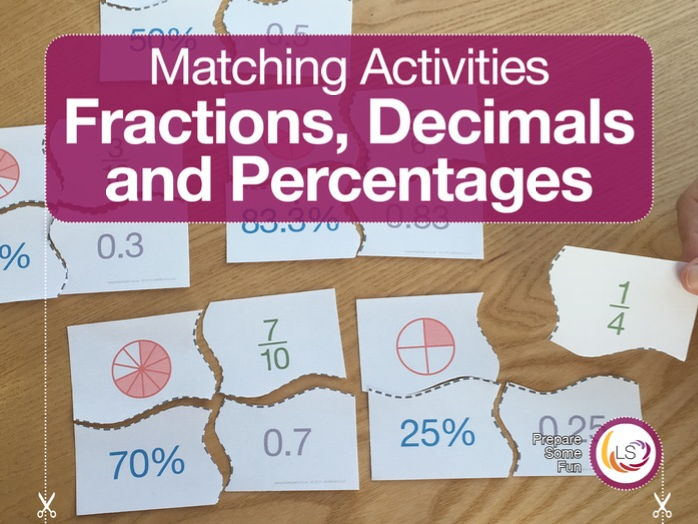#### Fraction, Decimal & Percentage | Matching Activity

(5)
This fraction, decimal and percentage matching puzzle is a fun way to test students ability convert between fractions, decimals and percentages. It contains 32 puzzles including all base fractions up to nine-tenths, and also includes a bonus ten-tenths to test understanding of a whole. It is desig...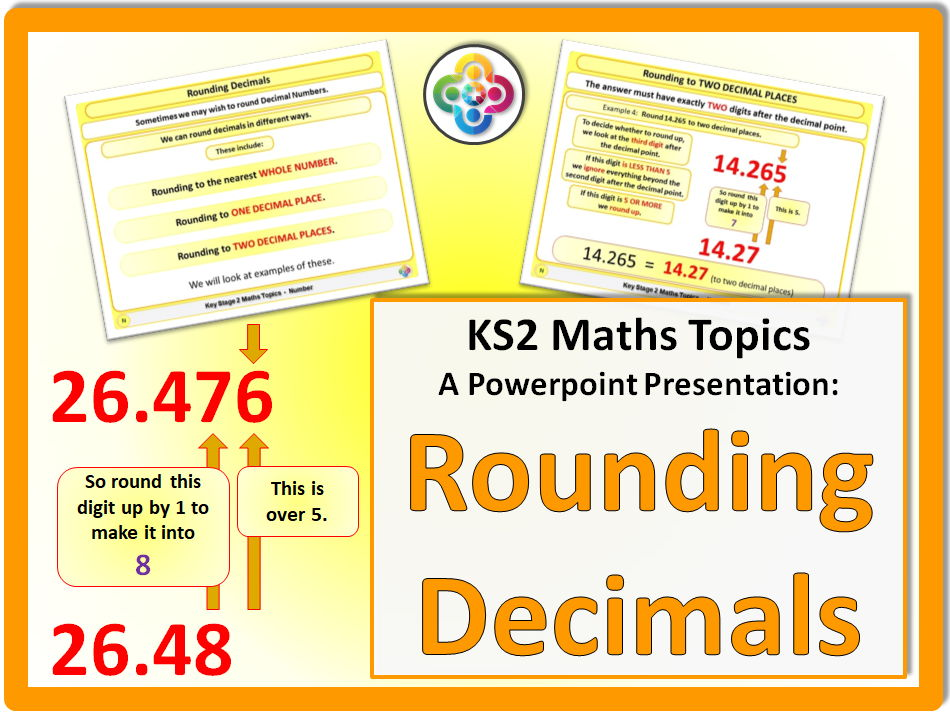#### Rounding Decimals KS2

(1)
A bright and stimulating Powerpoint to explain Rounding Decimals. This powerpoint has 18 slides (Including one title and one end slide). Designed for KS2. This covers: Rounding Decimals Rounding to the Nearest Whole Number Rounding to One Decimal Place Rounding to Two Decimal Places Included are...#### KS2/KS3 Maths: Place Value and Ordering Decimals

(1)
1\. Includes a PowerPoint and a worksheet designed to allow all pupils make progress and grain a full understanding of decimal place value. 2\. Decimal place value: Tenths, hundredths and thousandths. 3\. Highly visual approach to teaching and learning decimal place value.

### Four operations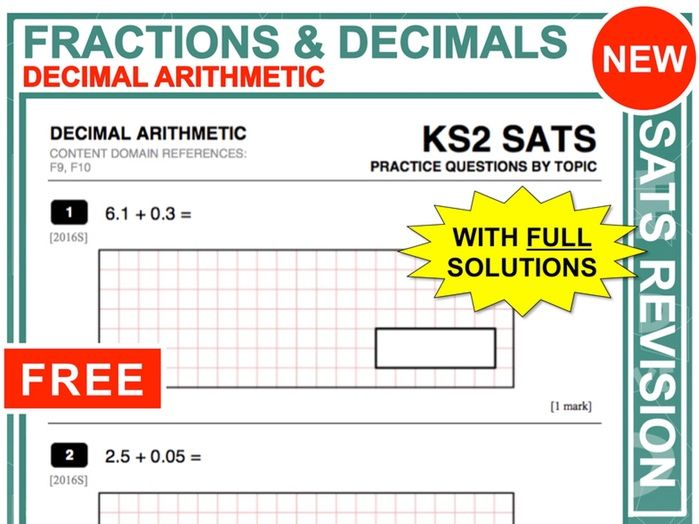#### KS2 Maths (Decimal Arithmetic)

(18)
These topic-focused SATs questions at the end of a unit will help to test and extend students' understanding as well as helping them to prepare for SATs next year. These questions have **fully-worked solutions** which can be displayed on a whiteboard making feedback with students more efficient. Cli...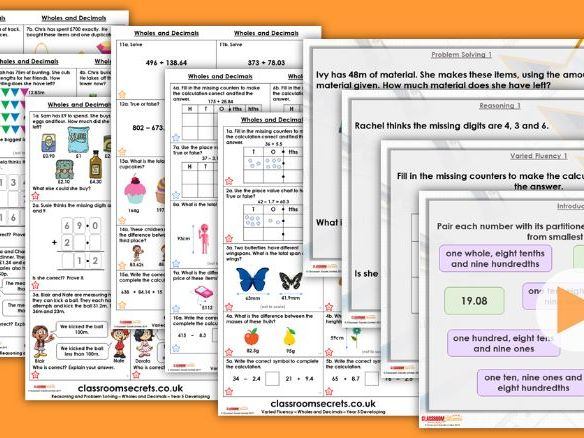#### Year 5 Adding and Subtracting Wholes and Decimals Summer Block 1 Step 9 Maths Lesson Pack

(1)
This Year 5 Adding and Subtracting Wholes and Decimals maths lesson pack includes a teaching PowerPoint and differentiated varied fluency, and reasoning problem solving questions. The varied fluency resource is differentiated in three ways and includes a selection of different questions types. The ...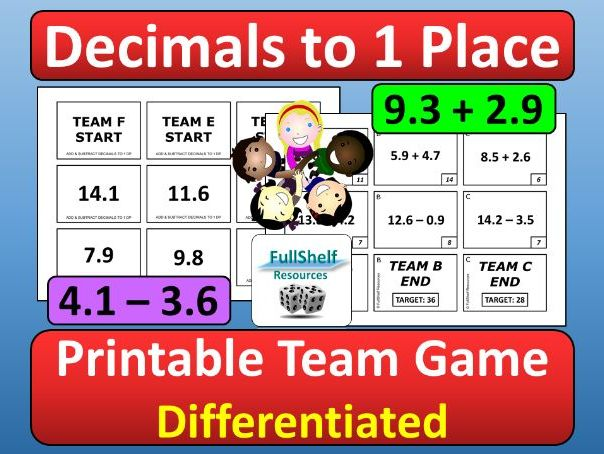#### Adding and Subtracting Decimals Game

(0)
Decimal Numbers to 1 Decimal Place Numeracy treasure hunt / scavenger hunt activity: an active printable mental maths team game, with **differentiation**, ideal as a whole class starter or plenary at KS2 (originally designed for Year 5 and 6), especially when focusing on addition and subtraction of ...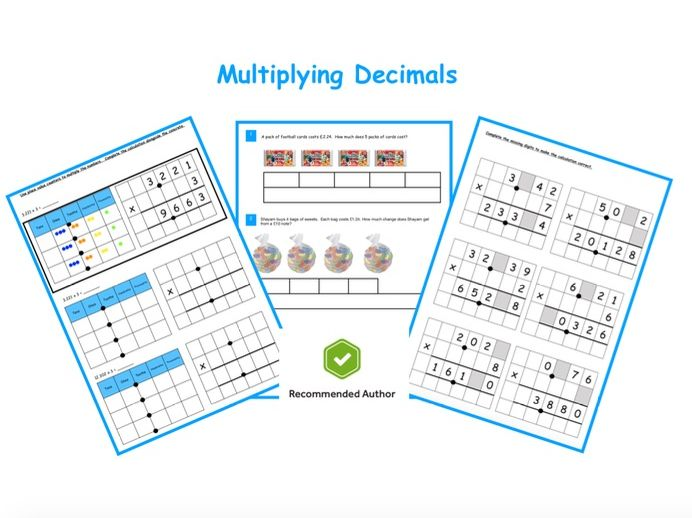#### Multiplying Decimals

(1)
Based on White Rose Maths Scheme and TES recommended. Included in this pack is: 3 worksheets based on the White Rose Schemes varied fluency and reasoning questions - including representing the question as a visual, missing digits and worded problems. 4 differentiated worksheets on multiplying...